### Home > MC2 > Chapter 7 > Lesson 7.1.2 > Problem7-20

7-20.
1. For each equation below, solve for x. Sometimes the easiest strategy is to use mental math. Homework Help ✎

1.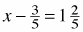2.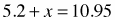3.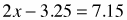4.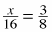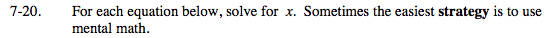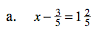$\text{What does } \frac{3}{5} + \frac{2}{5} = ?$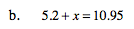x = 5.75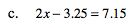x = 5.2
Be sure to know how to get this answer using mental math. Recognize that 3.25 + 7.15 equals an even decimal, 10.4.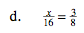8(2) = 16
What must x be?